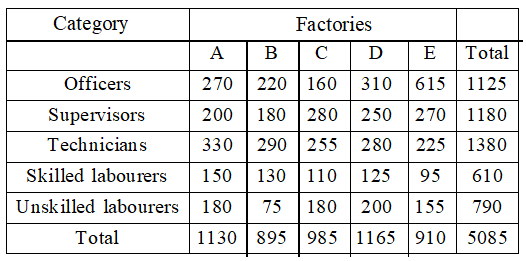Following table given the number of employees in different categories in five factories A, B, C, D and E. Study the table carefully and answer the questions given below.          The total strength of factory C in approximately by what percent more as compared to that of f    actory B?

# Following table given the number of employees in different categories in five factories A, B, C, D and E. Study the table carefully and answer the questions given below.The total strength of factory C in approximately by what percent more as compared to that of f    actory B?

1. A

5%

2. B

8%

3. C

20%

4. D

10%

Fill Out the Form for Expert Academic Guidance!l

+91

Live ClassesBooksTest SeriesSelf Learning

Verify OTP Code (required)

### Solution:

$\begin{array}{l}\frac{\left(985-895\right)}{895}\\ \left(\frac{90}{895}\right)×100=\text{\hspace{0.17em}}Approc\text{\hspace{0.17em}\hspace{0.17em}}10%\end{array}$+91

Live ClassesBooksTest SeriesSelf Learning

Verify OTP Code (required)